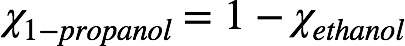# Problem: At 35°C the vapor pressure of pure ethanol (C2H5OH, M = 46.1 g/mol) is pE° = 100.0 torr, and the vapor pressure of pure 1-propanol (C3H7OH, M = 60.1 g/mol) is pP° = 37.6 torr.  A solution at equilibrium at this temperature has a total pressure p = 84.2 torr.  Assuming the solution is ideal, what is the partial pressure of ethanol above the solution?

###### FREE Expert Solution
87% (154 ratings)
###### FREE Expert Solution

We’re being asked to determine the partial pressure of ethanol (C2H5OH) above a solution. Recall that the vapor pressure of a solution can be given by Raoult’s Law.

The given solutions are composed of ethanol and 1-propanol, which are both volatile. For a solution with volatile solute and solvent, Raoult’s Law is given as:where P˚ = vapor pressure of pure component and χ = mole fraction of component. Recall that the mole fractions in a solution add up to 1. Since the solution is composed of ethanol and 1-propanol, this means:87% (154 ratings)###### Problem Details

At 35°C the vapor pressure of pure ethanol (C2H5OH, M = 46.1 g/mol) is pE° = 100.0 torr, and the vapor pressure of pure 1-propanol (C3H7OH, M = 60.1 g/mol) is pP° = 37.6 torr.  A solution at equilibrium at this temperature has a total pressure p = 84.2 torr.  Assuming the solution is ideal, what is the partial pressure of ethanol above the solution?

What scientific concept do you need to know in order to solve this problem?

Our tutors have indicated that to solve this problem you will need to apply the The Colligative Properties concept. You can view video lessons to learn The Colligative Properties. Or if you need more The Colligative Properties practice, you can also practice The Colligative Properties practice problems.

What is the difficulty of this problem?

Our tutors rated the difficulty ofAt 35°C the vapor pressure of pure ethanol (C2H5OH, M = 46.1...as medium difficulty.

How long does this problem take to solve?

Our expert Chemistry tutor, Jules took 6 minutes and 33 seconds to solve this problem. You can follow their steps in the video explanation above.

What professor is this problem relevant for?

Based on our data, we think this problem is relevant for Professor Ratliff's class at USF.